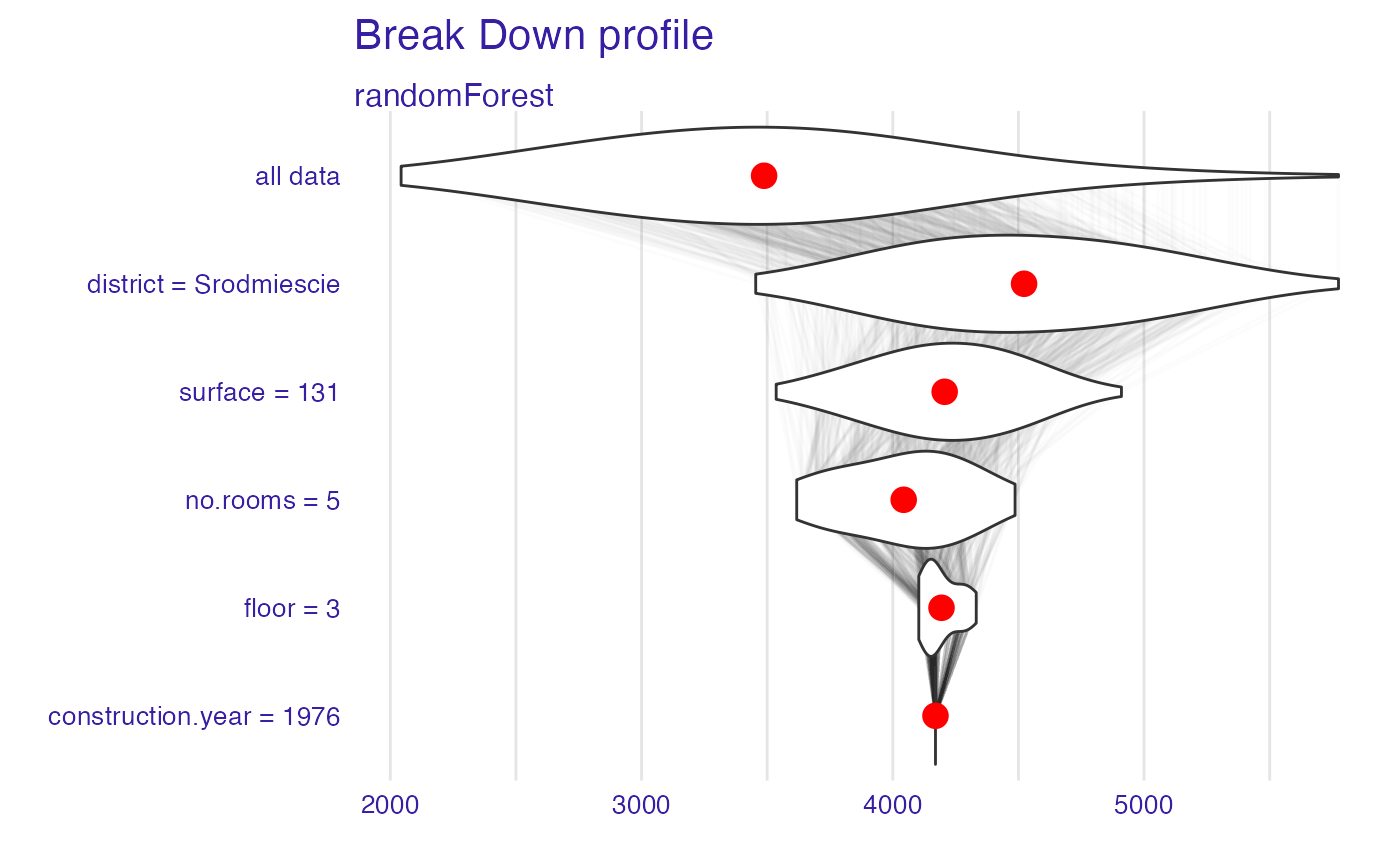This function finds Variable attributions via Sequential Variable Conditioning. The complexity of this function is O(2*p). This function works in a similar way to step-up and step-down greedy approximations in function break_down. The main difference is that in the first step the order of variables is determined. And in the second step the impact is calculated.

local_attributions(x, ...)

# S3 method for explainer
local_attributions(x, new_observation, keep_distributions = FALSE, ...)

# S3 method for default
x,
data,
predict_function = predict,
new_observation,
label = class(x),
keep_distributions = FALSE,
order = NULL,
...
)

## Arguments

x

an explainer created with function explain or a model.

...

other parameters.

new_observation

a new observation with columns that correspond to variables used in the model.

keep_distributions

if TRUE, then distribution of partial predictions is stored and can be plotted with the generic plot().

data

validation dataset, will be extracted from x if it is an explainer.

predict_function

predict function, will be extracted from x if it is an explainer.

label

name of the model. By default it's extracted from the 'class' attribute of the model.

order

if not NULL, then it will be a fixed order of variables. It can be a numeric vector or vector with names of variables.

## Value

an object of the break_down class.

break_down, local_interactions

## Examples

library("DALEX")
library("iBreakDown")
set.seed(1313)
model_titanic_glm <- glm(survived ~ gender + age + fare,
data = titanic_imputed, family = "binomial")
explain_titanic_glm <- explain(model_titanic_glm,
data = titanic_imputed,
y = titanic_imputed$survived, label = "glm") #> Preparation of a new explainer is initiated #> -> model label : glm #> -> data : 2207 rows 8 cols #> -> target variable : 2207 values #> -> predict function : yhat.glm will be used ( default ) #> -> predicted values : No value for predict function target column. ( default ) #> -> model_info : package stats , ver. 4.1.2 , task classification ( default ) #> -> predicted values : numerical, min = 0.1490412 , mean = 0.3221568 , max = 0.9878987 #> -> residual function : difference between y and yhat ( default ) #> -> residuals : numerical, min = -0.8898433 , mean = 4.198546e-13 , max = 0.8448637 #> A new explainer has been created! bd_glm <- local_attributions(explain_titanic_glm, titanic_imputed[1, ]) bd_glm #> contribution #> glm: intercept 0.322 #> glm: gender = male -0.107 #> glm: fare = 7.11 -0.018 #> glm: age = 42 -0.014 #> glm: class = 3rd 0.000 #> glm: embarked = Southampton 0.000 #> glm: sibsp = 0 0.000 #> glm: parch = 0 0.000 #> glm: survived = 0 0.000 #> glm: prediction 0.183 plot(bd_glm, max_features = 3)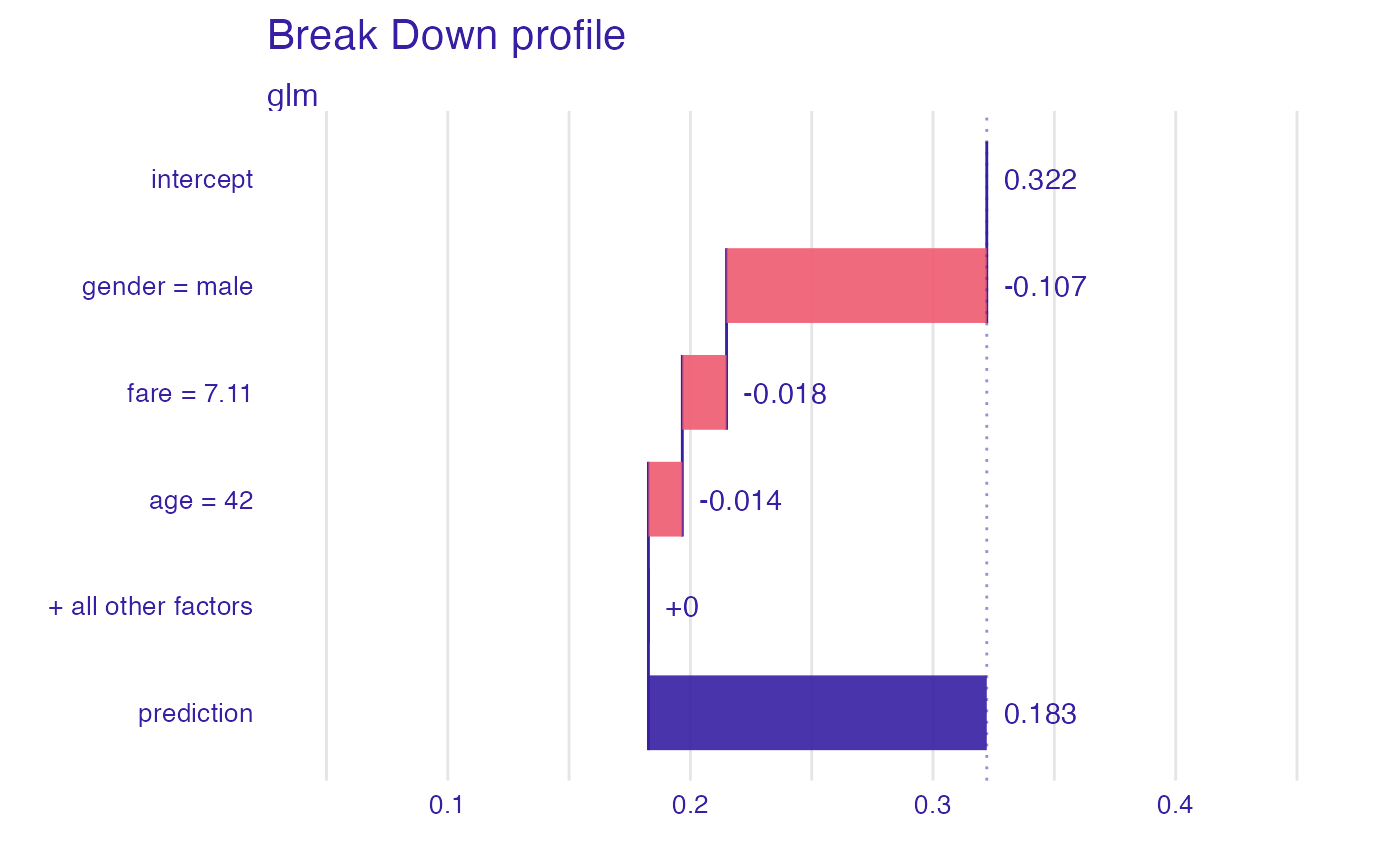# \dontrun{ ## Not run: library("randomForest") set.seed(1313) # example with interaction # classification for HR data model <- randomForest(status ~ . , data = HR) new_observation <- HR_test[1,] explainer_rf <- explain(model, data = HR[1:1000,1:5]) #> Preparation of a new explainer is initiated #> -> model label : randomForest ( default ) #> -> data : 1000 rows 5 cols #> -> target variable : not specified! ( WARNING ) #> -> predict function : yhat.randomForest will be used ( default ) #> -> predicted values : No value for predict function target column. ( default ) #> -> model_info : package randomForest , ver. 4.7.1 , task multiclass ( default ) #> -> model_info : Model info detected multiclass task but 'y' is a NULL . ( WARNING ) #> -> model_info : By deafult multiclass tasks supports only factor 'y' parameter. #> -> model_info : Consider changing to a factor vector with true class names. #> -> model_info : Otherwise I will not be able to calculate residuals or loss function. #> -> predicted values : predict function returns multiple columns: 3 ( default ) #> -> residual function : difference between 1 and probability of true class ( default ) #> A new explainer has been created! bd_rf <- local_attributions(explainer_rf, new_observation) bd_rf #> contribution #> randomForest.fired: intercept 0.386 #> randomForest.fired: hours = 42.32 0.231 #> randomForest.fired: evaluation = 2 0.062 #> randomForest.fired: salary = 2 -0.272 #> randomForest.fired: age = 57.73 0.092 #> randomForest.fired: gender = male 0.281 #> randomForest.fired: prediction 0.778 #> randomForest.ok: intercept 0.278 #> randomForest.ok: hours = 42.32 -0.053 #> randomForest.ok: evaluation = 2 0.091 #> randomForest.ok: salary = 2 0.271 #> randomForest.ok: age = 57.73 -0.086 #> randomForest.ok: gender = male -0.283 #> randomForest.ok: prediction 0.218 #> randomForest.promoted: intercept 0.336 #> randomForest.promoted: hours = 42.32 -0.178 #> randomForest.promoted: evaluation = 2 -0.152 #> randomForest.promoted: salary = 2 0.001 #> randomForest.promoted: age = 57.73 -0.006 #> randomForest.promoted: gender = male 0.002 #> randomForest.promoted: prediction 0.004 plot(bd_rf)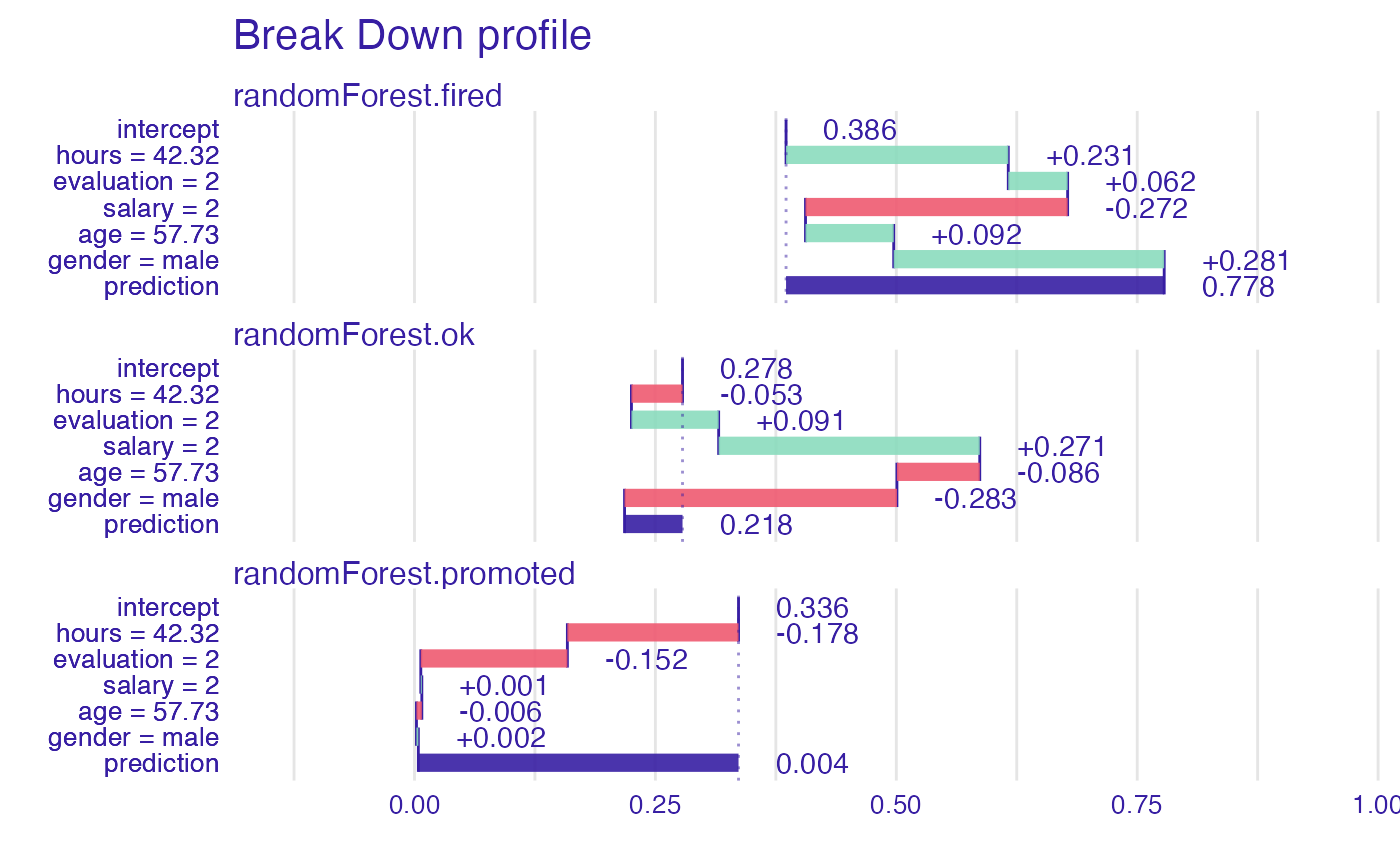plot(bd_rf, baseline = 0)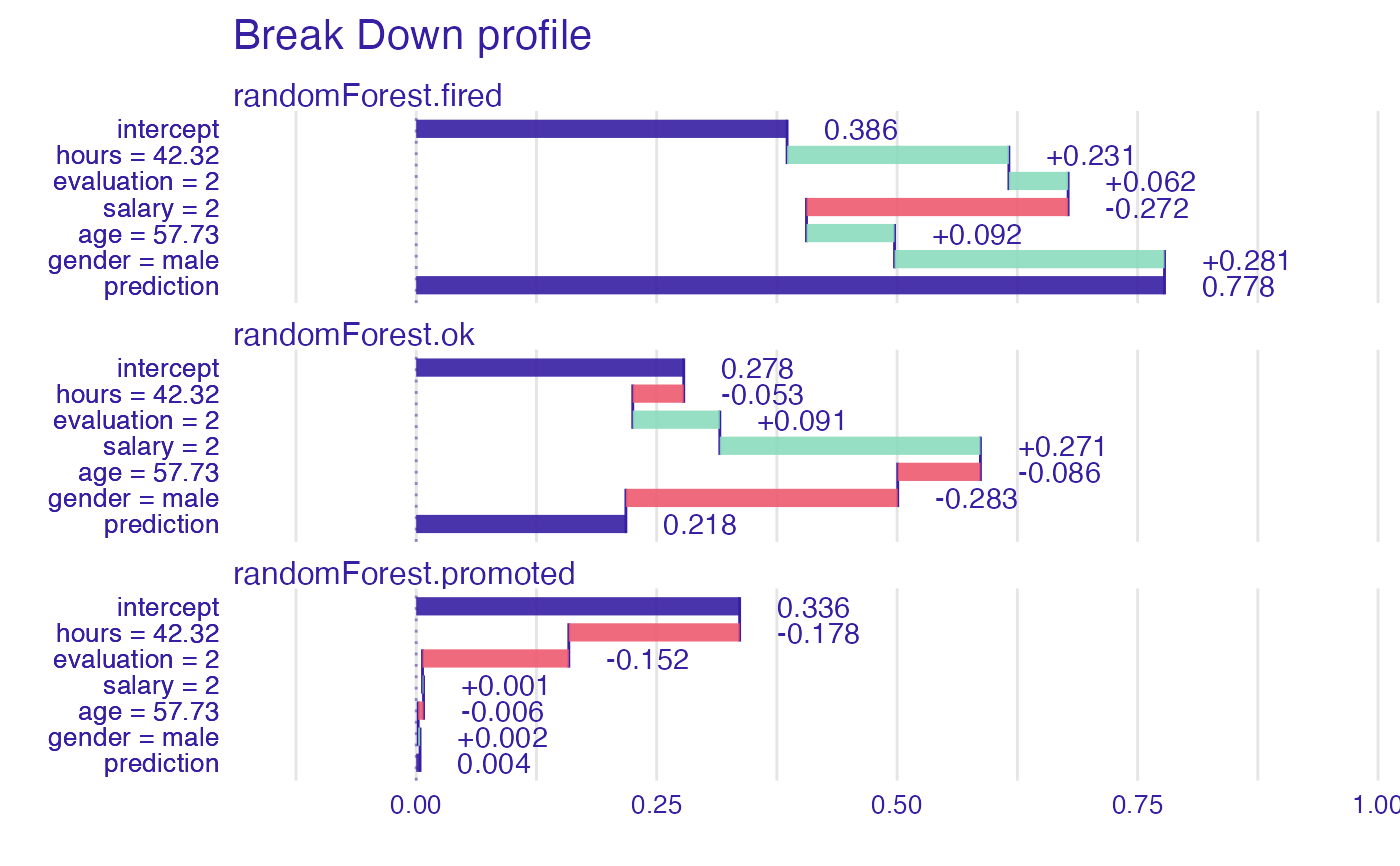# example for regression - apartment prices # here we do not have interactions model <- randomForest(m2.price ~ . , data = apartments) explainer_rf <- explain(model, data = apartments_test[1:1000,2:6], y = apartments_test$m2.price[1:1000])
#> Preparation of a new explainer is initiated
#>   -> model label       :  randomForest  (  default  )
#>   -> data              :  1000  rows  5  cols
#>   -> target variable   :  1000  values
#>   -> predict function  :  yhat.randomForest  will be used (  default  )
#>   -> predicted values  :  No value for predict function target column. (  default  )
#>   -> model_info        :  package randomForest , ver. 4.7.1 , task regression (  default  )
#>   -> predicted values  :  numerical, min =  2043.066 , mean =  3487.722 , max =  5773.976
#>   -> residual function :  difference between y and yhat (  default  )
#>   -> residuals         :  numerical, min =  -630.6766 , mean =  1.057813 , max =  1256.239
#>   A new explainer has been created!

apartments_test[1,])
bd_rf
#>                                        contribution
#> randomForest: intercept                    3487.722
#> randomForest: district = Srodmiescie       1034.737
#> randomForest: surface = 131                -315.991
#> randomForest: no.rooms = 5                 -163.113
#> randomForest: floor = 3                     150.529
#> randomForest: construction.year = 1976      -24.021
#> randomForest: prediction                   4169.863
plot(bd_rf, digits = 1)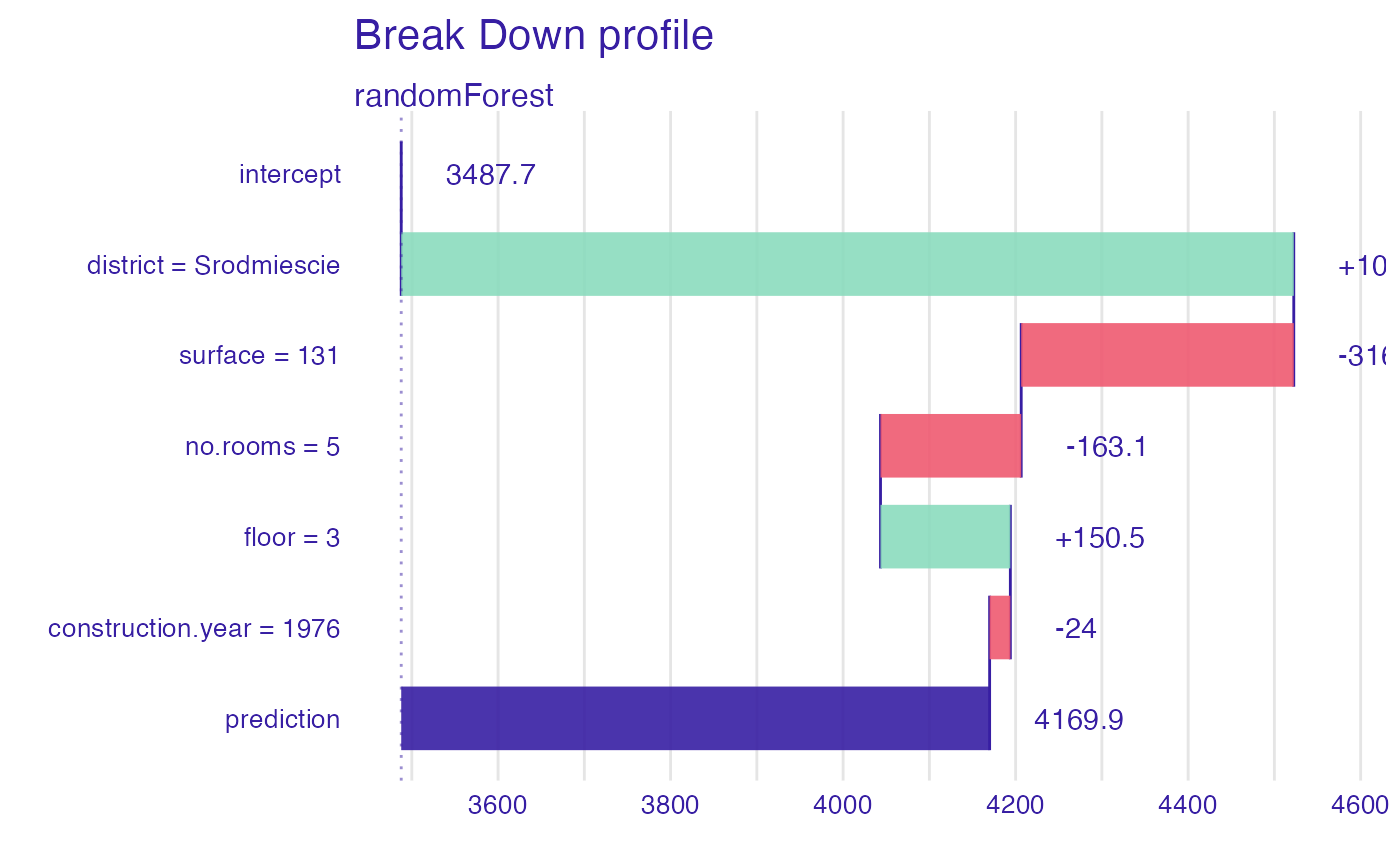#> Warning: fun.y is deprecated. Use fun instead.